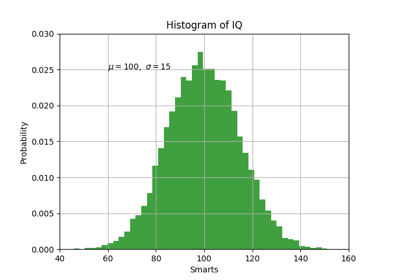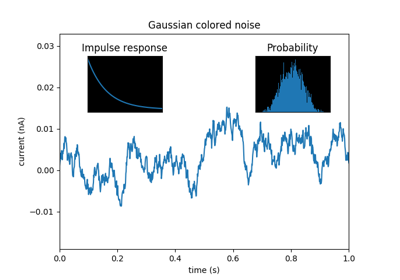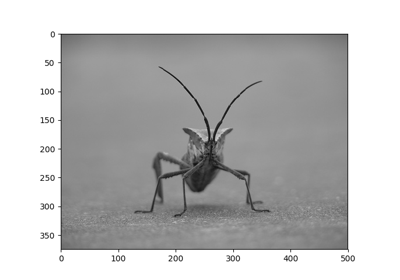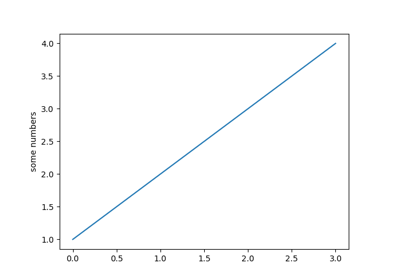# matplotlib.pyplot.hist¶

`matplotlib.pyplot.``hist`(x, bins=None, range=None, density=None, weights=None, cumulative=False, bottom=None, histtype='bar', align='mid', orientation='vertical', rwidth=None, log=False, color=None, label=None, stacked=False, normed=None, hold=None, data=None, **kwargs)[source]

Plot a histogram.

Compute and draw the histogram of x. The return value is a tuple (n, bins, patches) or ([n0, n1, …], bins, [patches0, patches1,…]) if the input contains multiple data.

Multiple data can be provided via x as a list of datasets of potentially different length ([x0, x1, …]), or as a 2-D ndarray in which each column is a dataset. Note that the ndarray form is transposed relative to the list form.

Masked arrays are not supported at present.

Parameters: x : (n,) array or sequence of (n,) arrays Input values, this takes either a single array or a sequence of arrays which are not required to be of the same length bins : integer or sequence or ‘auto’, optional If an integer is given, `bins + 1` bin edges are calculated and returned, consistent with `numpy.histogram()`. If `bins` is a sequence, gives bin edges, including left edge of first bin and right edge of last bin. In this case, `bins` is returned unmodified. All but the last (righthand-most) bin is half-open. In other words, if `bins` is: ```[1, 2, 3, 4] ``` then the first bin is `[1, 2)` (including 1, but excluding 2) and the second `[2, 3)`. The last bin, however, is `[3, 4]`, which includes 4. Unequally spaced bins are supported if bins is a sequence. If Numpy 1.11 is installed, may also be `'auto'`. Default is taken from the rcParam `hist.bins`. range : tuple or None, optional The lower and upper range of the bins. Lower and upper outliers are ignored. If not provided, range is `(x.min(), x.max())`. Range has no effect if bins is a sequence. If bins is a sequence or range is specified, autoscaling is based on the specified bin range instead of the range of x. Default is `None` density : boolean, optional If `True`, the first element of the return tuple will be the counts normalized to form a probability density, i.e., the area (or integral) under the histogram will sum to 1. This is achieved by dividing the count by the number of observations times the bin width and not dividing by the total number of observations. If stacked is also `True`, the sum of the histograms is normalized to 1. Default is `None` for both normed and density. If either is set, then that value will be used. If neither are set, then the args will be treated as `False`. If both density and normed are set an error is raised. weights : (n, ) array_like or None, optional An array of weights, of the same shape as x. Each value in x only contributes its associated weight towards the bin count (instead of 1). If normed or density is `True`, the weights are normalized, so that the integral of the density over the range remains 1. Default is `None` cumulative : boolean, optional If `True`, then a histogram is computed where each bin gives the counts in that bin plus all bins for smaller values. The last bin gives the total number of datapoints. If normed or density is also `True` then the histogram is normalized such that the last bin equals 1. If cumulative evaluates to less than 0 (e.g., -1), the direction of accumulation is reversed. In this case, if normed and/or density is also `True`, then the histogram is normalized such that the first bin equals 1. Default is `False` bottom : array_like, scalar, or None Location of the bottom baseline of each bin. If a scalar, the base line for each bin is shifted by the same amount. If an array, each bin is shifted independently and the length of bottom must match the number of bins. If None, defaults to 0. Default is `None` histtype : {‘bar’, ‘barstacked’, ‘step’, ‘stepfilled’}, optional The type of histogram to draw. ‘bar’ is a traditional bar-type histogram. If multiple data are given the bars are arranged side by side. ‘barstacked’ is a bar-type histogram where multiple data are stacked on top of each other. ‘step’ generates a lineplot that is by default unfilled. ‘stepfilled’ generates a lineplot that is by default filled. Default is ‘bar’ align : {‘left’, ‘mid’, ‘right’}, optional Controls how the histogram is plotted. ‘left’: bars are centered on the left bin edges. ‘mid’: bars are centered between the bin edges. ‘right’: bars are centered on the right bin edges. Default is ‘mid’ orientation : {‘horizontal’, ‘vertical’}, optional If ‘horizontal’, `barh` will be used for bar-type histograms and the bottom kwarg will be the left edges. rwidth : scalar or None, optional The relative width of the bars as a fraction of the bin width. If `None`, automatically compute the width. Ignored if histtype is ‘step’ or ‘stepfilled’. Default is `None` log : boolean, optional If `True`, the histogram axis will be set to a log scale. If log is `True` and x is a 1D array, empty bins will be filtered out and only the non-empty `(n, bins, patches)` will be returned. Default is `False` color : color or array_like of colors or None, optional Color spec or sequence of color specs, one per dataset. Default (`None`) uses the standard line color sequence. Default is `None` label : string or None, optional String, or sequence of strings to match multiple datasets. Bar charts yield multiple patches per dataset, but only the first gets the label, so that the legend command will work as expected. default is `None` stacked : boolean, optional If `True`, multiple data are stacked on top of each other If `False` multiple data are arranged side by side if histtype is ‘bar’ or on top of each other if histtype is ‘step’ Default is `False` normed : bool, optional Deprecated; use the density keyword argument instead. n : array or list of arrays The values of the histogram bins. See normed or density and weights for a description of the possible semantics. If input x is an array, then this is an array of length nbins. If input is a sequence arrays `[data1, data2,..]`, then this is a list of arrays with the values of the histograms for each of the arrays in the same order. bins : array The edges of the bins. Length nbins + 1 (nbins left edges and right edge of last bin). Always a single array even when multiple data sets are passed in. patches : list or list of lists Silent list of individual patches used to create the histogram or list of such list if multiple input datasets. **kwargs : `Patch` properties

`hist2d`
2D histograms

Notes

Note

In addition to the above described arguments, this function can take a data keyword argument. If such a data argument is given, the following arguments are replaced by data[<arg>]:

• All arguments with the following names: ‘weights’, ‘x’.

## Examples using `matplotlib.pyplot.hist`¶Pyplot TextAxes DemoSVG HistogramImage tutorialPyplot tutorial